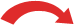# Convert Percents to Fractions

### To convert a Percent to a Fraction follow these steps:

• Step 1: Write down the percent divided by 100 like this: percent 100
• Step 2: If the percent is not a whole number, then multiply both top and bottom by 10 for every number after the decimal point. (For example, if there is one number after the decimal, then use 10, if there are two then use 100, etc.)
• Step 3: Simplify (or reduce) the fraction

### Example: Convert 11% to a fraction

Step 1: Write down:

11 100

Step 2: The percent is a whole number, go straight to step 3.

Step 3: The fraction cannot be simplified further.

### Example: Convert 75% to a fraction

Step 1: Write down:

75 100

Step 2: The percent is a whole number, go straight to step 3.

Step 3: Simplify the fraction (this took me two steps, you may be able to do it one!):

 ÷5 ÷ 575 100 = 15 20 = 34÷5 ÷ 5

Note: 75100 is called a decimal fraction and 34 is called a common fraction !

### Example: Convert 62.5% to a fraction

Step 1: Write down:

62.5 100

Step 2: Multiply both top and bottom by 10 (because there is 1 digit after the decimal place)

 × 1062.5 100 = 625 1000× 10

(See how this neatly makes the top a whole number?)

Step 3: Simplify the fraction (this took me two steps, you may be able to do it one!) :

 ÷ 25 ÷ 5625 1000 = 25 40 = 5 8÷ 25 ÷ 5

### Example: Convert 150% to a fraction

Step 1: Write down:

150 100

Step 2: The percent is a whole number, go straight to step 3.

Step 3: Simplify the fraction (I did it one step):

 ÷ 50150 100 = 3 2÷ 50